# Introduction to Big Data analysis with Spark

Hello, we’ll be introducing Spark in this series of articles. Spark can also be developed with many programming languages. We will use python in our series of articles.

# Introduction to Big Data analysis with Spark

Apache Spark provides high-level API’s in Scala, Java, Python and R. You will learn about PySpark which is Spark’s version of Python

• Apache Spark is written in Scala
• To support Python with Spark, Apache Spark Community released PySpark
• Similar computation speed and power as Scala.
• PySpark APIs are similar to Pandas and Scikit-learn

#### What is Spark shell

Spark comes with interactive shells that enable ad-hoc data analysis.  Spark shell is an interactive environment through which one can access Spark’s functionality quickly and conveniently.

#### PySpark Shell

• PySpark shell is the Python-based command line tool
• PySpark shell allows data scientists interface with Spark data structures.

## Introduction to Big Data analysis with Spark

Let’s do a little example. No matter what operating system you are using, it must be spark installed, If spark is not installed, you can find it here. https://phoenixnap.com/kb/install-spark-on-windows-10

Open cmd or terminal and start pyspark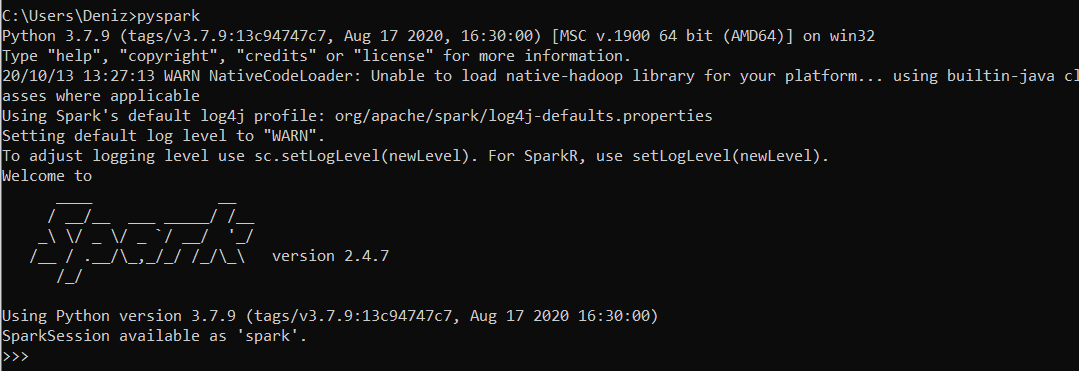Print the version of SparkContext

`sc.version`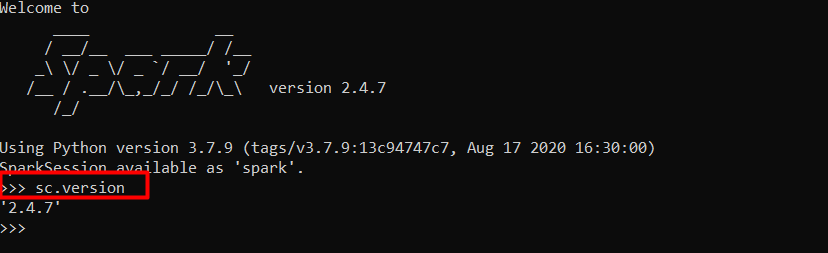Print the Python version of SparkContext

`sc.pythonVer`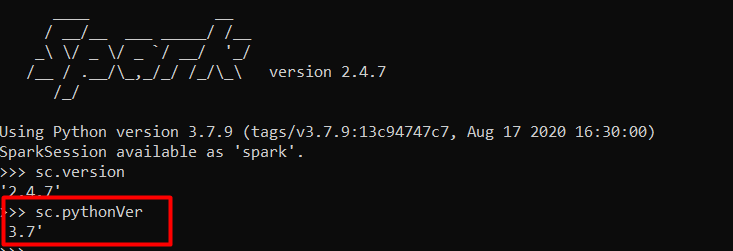Print the master of SparkContext

`sc.master`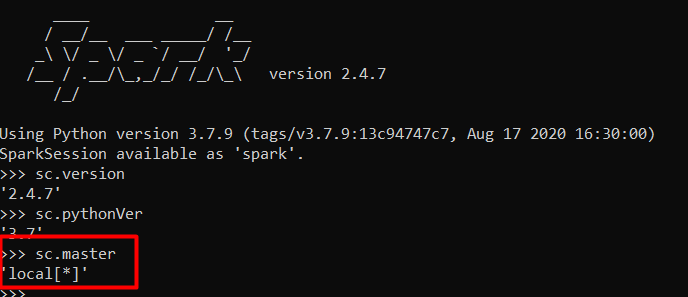# Introduction to Big Data analysis with Spark

The map() function in Python returns a list of the results after applying the given function to each item of a given iterable (list, tuple etc.). The general syntax of map() function is map(func,ite). We can also use lambda functions with map().

```my_list = range(1,10)

squared_list_lambda = list(map(lambda x: x**2, my_list))

print("The squared numbers are", squared_list_lambda)```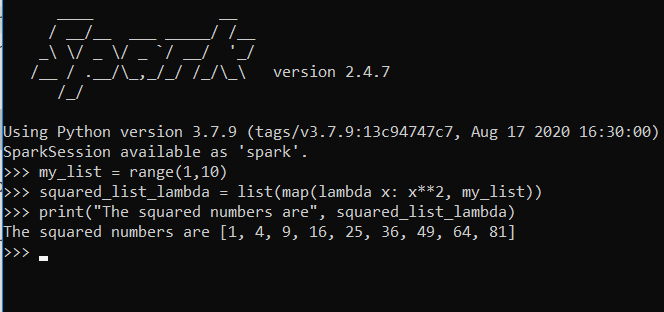Another function that is used extensively in Python is the filter() function. The filter() function in Python takes in a function and a list as arguments. The general syntax of the filter() function is filter(function, list_of_inputs). Similar to the map(), filter() can be used with lambda() function. The general syntax of the filter() function with lambda() is filter(lambda <argument>:<expression>, list).

```my_list2 = [10, 21, 31, 40, 51, 60, 72, 80, 93, 101]

filtered_list = list(filter(lambda x: (x%10 == 0), my_list2))

print("Numbers divisible by 10 are:", filtered_list)```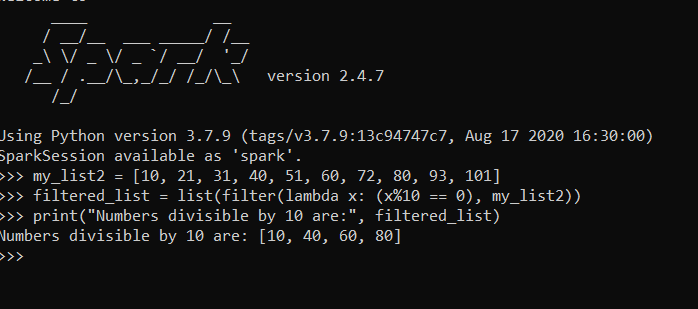See you in the next article.